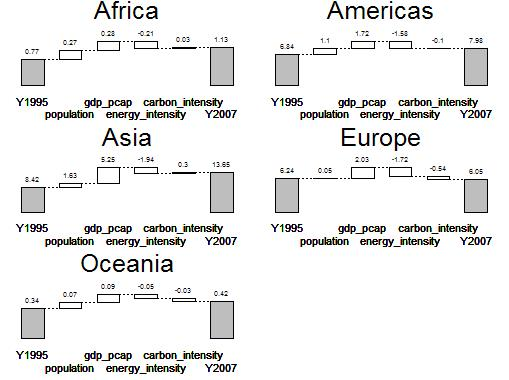## 2011-07-20

### Regional differences on what drives CO2 emissions

If you are investigating the change of CO2 emissions, then you might ask: Where do the changes occur? Well here is the answer.The staircase plots show the contributing factors to CO2 emissions for each continent. `population` refers to population effects, `gdp_pcap` refers to income per capita, `energy_intensity` refers to energy used per dollar added value, and `carbon intensity` refers to CO2 emissions per energy unit. The numbers are Gt CO2 and come, not very carefully curated, from Worldbank.

As you can see, europe’s emissions stay at nearly the same level, mostly because there is no population effect. On the other hand, Asia’s economic rise seems to explain much of the change of CO2 emissions in the time from 1995 to 2007.

Here is how to produce the chart:

First, load the library for index decomposition and the example data

``> library(ida)> contis <- transform_kaya("continent")``

Now we can create the plot using a split-apply approach

``> plot_one <- function(df) {+     decomp <- ida(df, effect = "variable", from = "Y1995", +         to = "Y2007", method = "lmdi1")+     conti <- df[1, "continent"]+     plot(decomp, digits = 2, stagger = TRUE, total.col = "gray", +         main = conti, cex.main = 2, mar = c(3, +             1, 3, 1) + 0.1)+ }> opar <- par(no.readonly = TRUE)> on.exit(par(opar))> par(mfrow = c(3, 2))> lapply(split(contis, contis\$continent), plot_one)``